In :
import numpy as np
import matplotlib.pyplot as plt
import csv
import pandas as pd
from IPython.display import Image
from scipy.integrate import odeint
from scipy.integrate import ode

%pylab inline

Populating the interactive namespace from numpy and matplotlib


1. Write a fourth-order fixed-time-step Runge-Kutta integrator from scratch. Inputs should consist of a starting time $t_0$, time step $\Delta t$, number of steps $n$, and starting value $\vec{x}(t_0)$ for the state vector. If your language allows functions as arguments, make the system derivative an argument as well. The output of this procedure - a series of state vectors representing the $n$-point state-space trajectory emenating from $\vec{x}(t_0)$ - should go to a file.

In :
def runge_kutta(f, t0, y0, dt, n, writecsv=False):
dim = y0.size

# Establish blank solution trajectory
# [[y00, ..., y0n, t0],
#  [y10, ..., y1n, t1],
# ...]
traj = np.zeros((n + 1, dim + 1), dtype=np.float64)

# Set initial position
traj[0, 0:dim] = y0
traj[0, -1]    = t0

# Iterate
for i in range(1, n + 1):
k1 = f(traj[i - 1, -1]           , traj[i - 1, 0:dim])
k2 = f(traj[i - 1, -1] + (dt / 2), traj[i - 1, 0:dim] + (k1 * dt / 2))
k3 = f(traj[i - 1, -1] + (dt / 2), traj[i - 1, 0:dim] + (k2 * dt / 2))
k4 = f(traj[i - 1, -1] + dt      , traj[i - 1, 0:dim] + k3 * dt)

# y
traj[i, 0:dim] = (traj[i - 1, 0:dim] + dt *
((k1 / 6) + (k2 / 3) +
(k3 / 3) + (k4 / 6)))
# t
traj[i, -1] = traj[i - 1, -1] + dt

if writecsv:
with open('rungekuttasteps.csv', 'w') as f:
csvwriter = csv.writer(f)
[csvwriter.writerow(line) for line in traj]
return traj


The rest of the problems in this set concern the following equation:

$$m l \theta^{\prime\prime}(t) + \beta l \theta^\prime(t) + mg \sin \theta (t) = A \cos(\alpha t)$$

This is the equation of motion of a forced, damped pendulum. The state vetor is ${[\theta, \omega]}^T$; the former is in radians, while the latter is in rad/s. Use your RK4 solver.

2. Use $m=0.1kg$, $l = 0.1m$, $\beta = 0$, and set the drive amplitude and frequency to zero ($\alpha = A = 0$).

(a) Turn in a plot of the state-space trajectory emanating from the point $[\theta, \omega] = [3, 0.1]$ with $\Delta t = 0.005$. Is this initial condition neat an equilibrium point? Which one? Is that point stable or unstable?

We first rewrite our equation as the following using the substitutions $\theta^{\prime\prime} = P(\theta, \omega, t)$, and $\theta^\prime = \omega$.

Note that $\theta$ and its derivatives are functions of time, however for this first part time remains constant and is therefore ignored.

$$P(\theta, \omega, t) = \frac{A \cos(\alpha t)}{ml} - \frac{\beta \omega}{m} - \frac{g \sin(\theta)}{l}$$
In :
m     = 0.1
l     = 0.1
b     = 0.0
alpha = 0.0
A     = 0.0
g     = 9.8

# [Angle, Speed]
initial_state = np.array([3, 0.1], dtype=np.float64)
dt = 0.005

# y is a two-parameter array [theta, omega]
pendulum = lambda t, y: np.array([y,
(((A * np.cos(alpha * t)) / (m * l)) -
((b * y) / m) -
((g * np.sin(y)) / l))])

data = runge_kutta(pendulum, 0, initial_state, dt, 1000)

fig = plt.figure()
ax = fig.add_axes([0.1, 0.1, 0.8, 0.8])
ax.plot(data[:, 0], data[:, 1])
ax.plot(np.arange(-5, 5, 1), np.zeros(10), 'k-')
ax.plot(np.zeros(50), np.arange(-25, 25, 1), 'k-')
plt.xlabel('Angle')
plt.ylabel('Speed')
plt.show()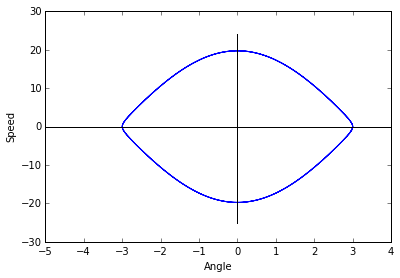Please reference Appendix (A) as well. I've used online code to sanity check my results, as well as built in libraries.

Our initial point (according to the solver) is on a stable orbit.

(B) Turn in a plot of the state-space trajectory emanating from the point $[\theta, \omega] = [0.01, 0]$. Does this trajectory look more like a perfect ellipse than the trajectory of part (a)?

In :
m     = 0.1
l     = 0.1
b     = 0.0
alpha = 0.0
A     = 0.0
g     = 9.8

# [Angle, Speed]
initial_state = np.array([0.1, 0], dtype=np.float64)
dt = 0.005

# y is a two-parameter array [theta, omega]
pendulum = lambda t, y: np.array([y,
(((A * np.cos(alpha * t)) / (m * l)) -
((b * y) / m) -
((g * np.sin(y)) / l))])

data = runge_kutta(pendulum, 0, initial_state, dt, 1000)

fig = plt.figure()
ax = fig.add_axes([0.1, 0.1, 0.8, 0.8])
ax.plot(data[:, 0], data[:, 1])
ax.plot(np.arange(-1, 1, 0.1), np.zeros(20), 'k-')
ax.plot(np.zeros(24), np.arange(-1.2, 1.2, 0.1), 'k-')
plt.xlabel('Angle')
plt.ylabel('Speed')
plt.show()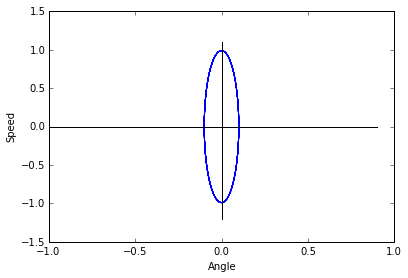This graph is also on a stable orbit, and does in fact appear to be a more perfect ellipse than in part (a).

3. Use your integrator to generate a state-space portrait of the system, using the coefficient values given in problem 2.

In :
m     = 0.1
l     = 0.1
b     = 0.0
alpha = 0.0
A     = 0.0
g     = 9.8
dt    = 0.005

# [Angle, Speed]
ti = np.arange(-5, 6, 0.5)
oi = np.arange(-8, 8, 0.5)
initial_states = []

for t in ti:
for o in oi:
initial_states.append(np.array([t, o], dtype=np.float64))

# y is a two-parameter array [theta, omega]
pendulum = lambda t, y: np.array([y,
(((A * np.cos(alpha * t)) / (m * l)) -
((b * y) / m) -
((g * np.sin(y)) / l))])

fig = plt.figure(figsize=(10, 10))
ax = fig.add_axes([0.1, 0.1, 0.8, 0.8])
for state in initial_states:
data = runge_kutta(pendulum, 0, state, dt, 130)
ax.plot(data[:, 0], data[:, 1])

ax.plot(np.arange(-5, 5, 1), np.zeros(10), 'k-')
ax.plot(np.zeros(10), np.arange(-5, 5, 1), 'k-')
plt.xlabel('Angle')
plt.ylabel('Speed')
plt.show()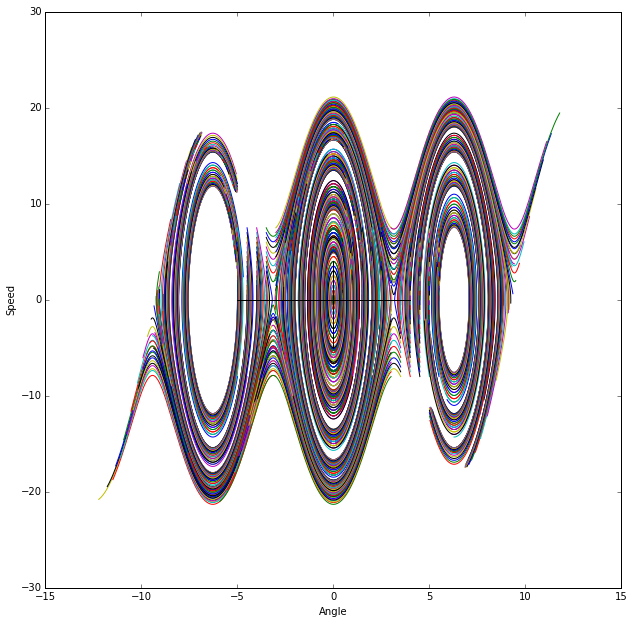4. Now repeat problem 3 with $\beta = 0.25$. What happens to the various features of the plot? What does this imply about the physical dynamics? Turn in a copy of this plot. What do you think would happen with higher or lower $\beta$s?

In :
m     = 0.1
l     = 0.1
b     = 0.25
alpha = 0.0
A     = 0.0
g     = 9.8
dt    = 0.005

# [Angle, Speed]
ti = np.arange(-6, 7, 0.5)
oi = np.arange(-6, 7, 0.5)
initial_states = []

for t in ti:
for o in oi:
initial_states.append(np.array([t, o], dtype=np.float64))

# y is a two-parameter array [theta, omega]
pendulum = lambda t, y: np.array([y,
(((A * np.cos(alpha * t)) / (m * l)) -
((b * y) / m) -
((g * np.sin(y)) / l))])

fig = plt.figure(figsize=(10, 10))
ax = fig.add_axes([0.1, 0.1, 0.8, 0.8])
for state in initial_states:
data = runge_kutta(pendulum, 0, state, dt, 200)
ax.plot(data[:, 0], data[:, 1])

ax.plot(np.arange(-5, 5, 1), np.zeros(10), 'k-')
ax.plot(np.zeros(10), np.arange(-5, 5, 1), 'k-')
plt.xlabel('Angle')
plt.ylabel('Speed')
plt.show()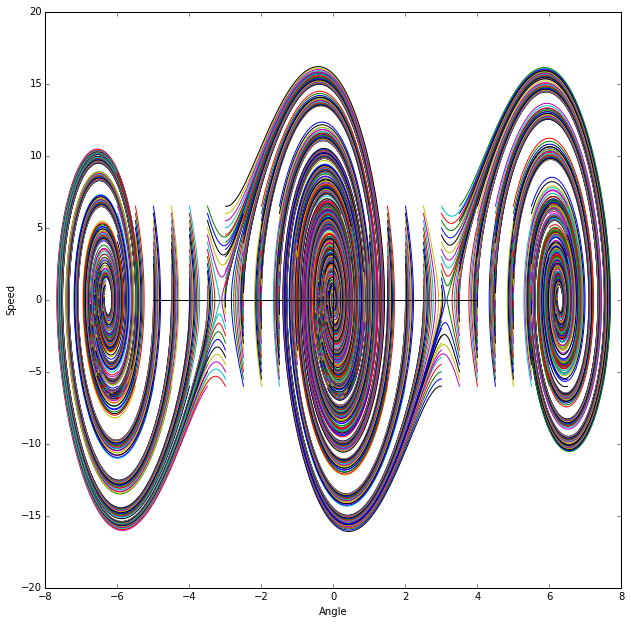This increases the acceleration making the speed change at a higher pace. Phrasing differentially, it elongates the ellipses vertically. A higher $\beta$ would elongate these further, while a lower $\beta$ (I think) would squish them down towards the $\theta$-axis.

5. Modify your code so that it plots $\theta \mod 2 \pi$ and see what that does to your results in problem 4.

In :
m     = 0.1
l     = 0.1
b     = 0.25
alpha = 0.0
A     = 0.0
g     = 9.8
dt    = 0.005

# [Angle, Speed]
ti = np.arange(-5, 6, 1)
oi = np.arange(-5, 6, 1)
initial_states = []

for t in ti:
for o in oi:
initial_states.append(np.array([t, o], dtype=np.float64))

# y is a two-parameter array [theta, omega]
pendulum = lambda t, y: np.array([y,
(((A * np.cos(alpha * t)) / (m * l)) -
((b * y) / m) -
((g * np.sin(y)) / l))])
fig = plt.figure()
ax = fig.add_axes([0.1, 0.1, 0.8, 0.8])
for state in initial_states:
data        = runge_kutta(pendulum, 0, state, dt, 200)
data[:, 0] %= 2 * np.pi
ax.scatter(data[:, 0], data[:, 1], s=1)

ax.plot(np.arange(0, 5, 1), np.zeros(5), 'k-')
ax.plot(np.zeros(5), np.arange(0, 5, 1), 'k-')
plt.xlabel('Angle')
plt.ylabel('Speed')
plt.show()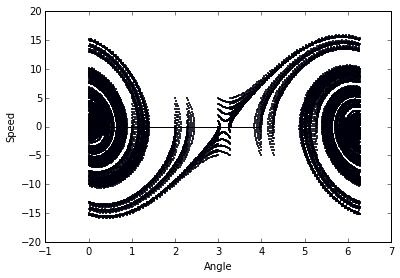It overlays one side onto the other, as is expected. The horizontal lines are a result of the plotter not understanding that some jump like $[\ldots, 2 \pi, 0, \ldots]$ is not to be graphed. This modulo graphing also demonstrates a deeper pattern in the system.

6. Leaving $\beta = 0.25$, turn on the drive. Vary the drive frequency $\alpha$ and amplitude $A$ and describe and explain what you see on the plots (using chaotic vocabulary). Find a chaotic trajectory and turn in a plot. Make sure you plot $\theta \mod 2 \pi$.

In :
m     = 0.1
l     = 0.1
b     = 0.25
alpha = 1
A     = 2
g     = 9.8
dt    = 0.005

# [Angle, Speed]
ti = np.arange(-5, 6, 0.5)
oi = np.arange(-5, 6, 0.5)
initial_states = []

for t in ti:
for o in oi:
initial_states.append(np.array([t, o], dtype=np.float64))

# y is a two-parameter array [theta, omega]
pendulum = lambda t, y: np.array([y,
(((A * np.cos(alpha * t)) / (m * l)) -
((b * y) / m) -
((g * np.sin(y)) / l))])
fig = plt.figure()
ax = fig.add_axes([0.1, 0.1, 0.8, 0.8])
for state in initial_states:
data = runge_kutta(pendulum, 0, state, dt, 100)
ax.scatter(data[:, 0] % (2 * np.pi), data[:, 1], s=1)

ax.plot(np.arange(0, 5, 1), np.zeros(5), 'k-')
ax.plot(np.zeros(10), np.arange(-5, 5, 1), 'k-')
plt.xlabel('Angle')
plt.ylabel('Speed')
plt.show()

fig = plt.figure(figsize=(10, 10))
ax = fig.add_axes([0.1, 0.1, 0.8, 0.8])
for state in initial_states:
data = runge_kutta(pendulum, 0, state, dt, 500)
ax.plot(data[:, 0], data[:, 1])

ax.plot(np.arange(-5, 5, 1), np.zeros(10), 'k-')
ax.plot(np.zeros(10), np.arange(-5, 5, 1), 'k-')
plt.xlabel('Angle')
plt.ylabel('Speed')
plt.show()